Linear Regression and Correlation

# 68 The Correlation Coefficient r

As we begin this section we note that the type of data we will be working with has changed. Perhaps unnoticed, all the data we have been using is for a single variable. It may be from two samples, but it is still a univariate variable. The type of data described in the examples above and for any model of cause and effect is bivariate data — “bi” for two variables. In reality, statisticians use multivariate data, meaning many variables.

For our work we can classify data into three broad categories, time series data, cross-section data, and panel data. We met the first two very early on. Time series data measures a single unit of observation; say a person, or a company or a country, as time passes. What are measured will be at least two characteristics, say the person’s income, the quantity of a particular good they buy and the price they paid. This would be three pieces of information in one time period, say 1985. If we followed that person across time we would have those same pieces of information for 1985,1986, 1987, etc. This would constitute a times series data set. If we did this for 10 years we would have 30 pieces of information concerning this person’s consumption habits of this good for the past decade and we would know their income and the price they paid.

A second type of data set is for cross-section data. Here the variation is not across time for a single unit of observation, but across units of observation during one point in time. For a particular period of time we would gather the price paid, amount purchased, and income of many individual people.

A third type of data set is panel data. Here a panel of units of observation is followed across time. If we take our example from above we might follow 500 people, the unit of observation, through time, ten years, and observe their income, price paid and quantity of the good purchased. If we had 500 people and data for ten years for price, income and quantity purchased we would have 15,000 pieces of information. These types of data sets are very expensive to construct and maintain. They do, however, provide a tremendous amount of information that can be used to answer very important questions. As an example, what is the effect on the labor force participation rate of women as their family of origin, mother and father, age? Or are there differential effects on health outcomes depending upon the age at which a person started smoking? Only panel data can give answers to these and related questions because we must follow multiple people across time. The work we do here however will not be fully appropriate for data sets such as these.

Beginning with a set of data with two independent variables we ask the question: are these related? One way to visually answer this question is to create a scatter plot of the data. We could not do that before when we were doing descriptive statistics because those data were univariate. Now we have bivariate data so we can plot in two dimensions. Three dimensions are possible on a flat piece of paper, but become very hard to fully conceptualize. Of course, more than three dimensions cannot be graphed although the relationships can be measured mathematically.

To provide mathematical precision to the measurement of what we see we use the correlation coefficient. The correlation tells us something about the co-movement of two variables, but nothing about why this movement occurred. Formally, correlation analysis assumes that both variables being analyzed are independent variables. This means that neither one causes the movement in the other. Further, it means that neither variable is dependent on the other, or for that matter, on any other variable. Even with these limitations, correlation analysis can yield some interesting results.

The correlation coefficient, ρ (pronounced rho), is the mathematical statistic for a population that provides us with a measurement of the strength of a linear relationship between the two variables. For a sample of data, the statistic, r, developed by Karl Pearson in the early 1900s, is an estimate of the population correlation and is defined mathematically as: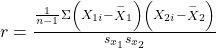OR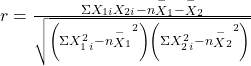where sx1 and sx2 are the standard deviations of the two independent variables X1 and X2,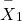and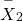are the sample means of the two variables, and X1i and X2i are the individual observations of X1 and X2. The correlation coefficient r ranges in value from -1 to 1. The second equivalent formula is often used because it may be computationally easier. As scary as these formulas look they are really just the ratio of the covariance between the two variables and the product of their two standard deviations. That is to say, it is a measure of relative variances.

In practice all correlation and regression analysis will be provided through computer software designed for these purposes. Anything more than perhaps one-half a dozen observations creates immense computational problems. It was because of this fact that correlation, and even more so, regression, were not widely used research tools until after the advent of “computing machines”. Now the computing power required to analyze data using regression packages is deemed almost trivial by comparison to just a decade ago.

To visualize any linear relationship that may exist review the plot of a scatter diagrams of the standardized data. (Figure) presents several scatter diagrams and the calculated value of r. In panels (a) and (b) notice that the data generally trend together, (a) upward and (b) downward. Panel (a) is an example of a positive correlation and panel (b) is an example of a negative correlation, or relationship. The sign of the correlation coefficient tells us if the relationship is a positive or negative (inverse) one. If all the values of X1 and X2 are on a straight line the correlation coefficient will be either 1 or -1 depending on whether the line has a positive or negative slope and the closer to one or negative one the stronger the relationship between the two variables. BUT ALWAYS REMEMBER THAT THE CORRELATION COEFFICIENT DOES NOT TELL US THE SLOPE.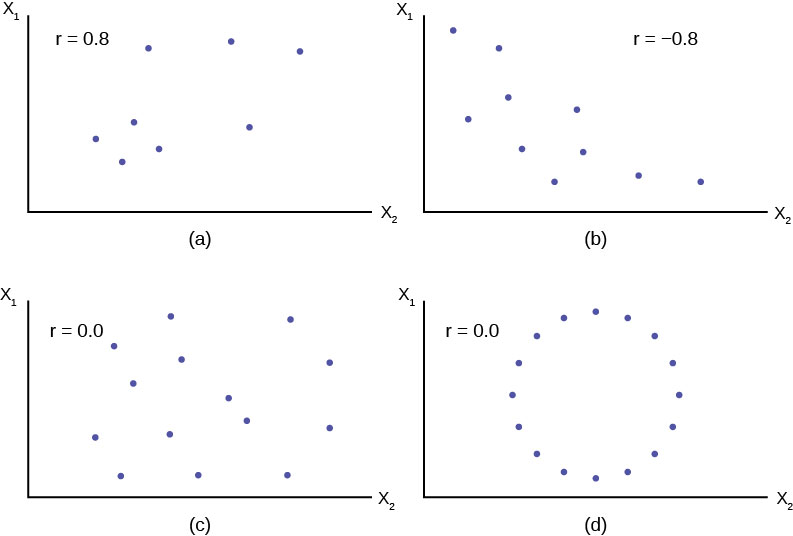Remember, all the correlation coefficient tells us is whether or not the data are linearly related. In panel (d) the variables obviously have some type of very specific relationship to each other, but the correlation coefficient is zero, indicating no linear relationship exists.

If you suspect a linear relationship between X1 and X2 then r can measure how strong the linear relationship is.

What the VALUE of r tells us:
• The value of r is always between –1 and +1: –1 ≤ r ≤ 1.
• The size of the correlation r indicates the strength of the linear relationship between X1 and X2. Values of r close to –1 or to +1 indicate a stronger linear relationship between X1 and X2.
• If r = 0 there is absolutely no linear relationship between X1 and X2(no linear correlation).
• If r = 1, there is perfect positive correlation. If r = –1, there is perfect negative correlation. In both these cases, all of the original data points lie on a straight line: ANY straight line no matter what the slope. Of course, in the real world, this will not generally happen.
What the SIGN of r tells us
• A positive value of r means that when X1 increases, X2 tends to increase and when X1 decreases, X2 tends to decrease (positive correlation).
• A negative value of r means that when X1 increases, X2 tends to decrease and when X1 decreases, X2 tends to increase (negative correlation).
Note

Strong correlation does not suggest that X1 causes X2 or X2 causes X1. We say “correlation does not imply causation.”

In order to have a correlation coefficient between traits A and B, it is necessary to have:

1. one group of subjects, some of whom possess characteristics of trait A, the remainder possessing those of trait B
2. measures of trait A on one group of subjects and of trait B on another group
3. two groups of subjects, one which could be classified as A or not A, the other as B or not B
4. two groups of subjects, one which could be classified as A or not A, the other as B or not B

d

Define the Correlation Coefficient and give a unique example of its use.

A measure of the degree to which variation of one variable is related to variation in one or more other variables. The most commonly used correlation coefficient indicates the degree to which variation in one variable is described by a straight line relation with another variable.

Suppose that sample information is available on family income and Years of schooling of the head of the household. A correlation coefficient = 0 would indicate no linear association at all between these two variables. A correlation of 1 would indicate perfect linear association (where all variation in family income could be associated with schooling and vice versa).

If the correlation between age of an auto and money spent for repairs is +.90

1. 81% of the variation in the money spent for repairs is explained by the age of the auto
2. 81% of money spent for repairs is unexplained by the age of the auto
3. 90% of the money spent for repairs is explained by the age of the auto
4. none of the above

a. 81% of the variation in the money spent for repairs is explained by the age of the auto

Suppose that college grade-point average and verbal portion of an IQ test had a correlation of .40. What percentage of the variance do these two have in common?

1. 20
2. 16
3. 40
4. 80

b. 16

True or false? If false, explain why: The coefficient of determination can have values between -1 and +1.

The coefficient of determination is r··2 with 0 ≤ r··2 ≤ 1, since -1 ≤ r ≤ 1.

True or False: Whenever r is calculated on the basis of a sample, the value which we obtain for r is only an estimate of the true correlation coefficient which we would obtain if we calculated it for the entire population.

True

Under a “scatter diagram” there is a notation that the coefficient of correlation is .10. What does this mean?

1. plus and minus 10% from the means includes about 68% of the cases
2. one-tenth of the variance of one variable is shared with the other variable
3. one-tenth of one variable is caused by the other variable
4. on a scale from -1 to +1, the degree of linear relationship between the two variables is +.10

d. on a scale from -1 to +1, the degree of linear relationship between the two variables is +.10

The correlation coefficient for X and Y is known to be zero. We then can conclude that:

1. X and Y have standard distributions
2. the variances of X and Y are equal
3. there exists no relationship between X and Y
4. there exists no linear relationship between X and Y
5. none of these

d. there exists no linear relationship between X and Y

What would you guess the value of the correlation coefficient to be for the pair of variables: “number of man-hours worked” and “number of units of work completed”?

1. Approximately 0.9
2. Approximately 0.4
3. Approximately 0.0
4. Approximately -0.4
5. Approximately -0.9

Approximately 0.9

In a given group, the correlation between height measured in feet and weight measured in pounds is +.68. Which of the following would alter the value of r?

1. height is expressed centimeters.
2. weight is expressed in Kilograms.
3. both of the above will affect r.
4. neither of the above changes will affect r.

d. neither of the above changes will affect r.

### Key Terms

Bivariate
two variables are present in the model where one is the “cause” or independent variable and the other is the “effect” of dependent variable.
Multivariate
a system or model where more than one independent variable is being used to predict an outcome. There can only ever be one dependent variable, but there is no limit to the number of independent variables.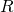– Correlation Coefficient
A number between −1 and 1 that represents the strength and direction of the relationship between “X” and “Y.” The value for “r” will equal 1 or −1 only if all the plotted points form a perfectly straight line.
Linear
a model that takes data and regresses it into a straight line equation.